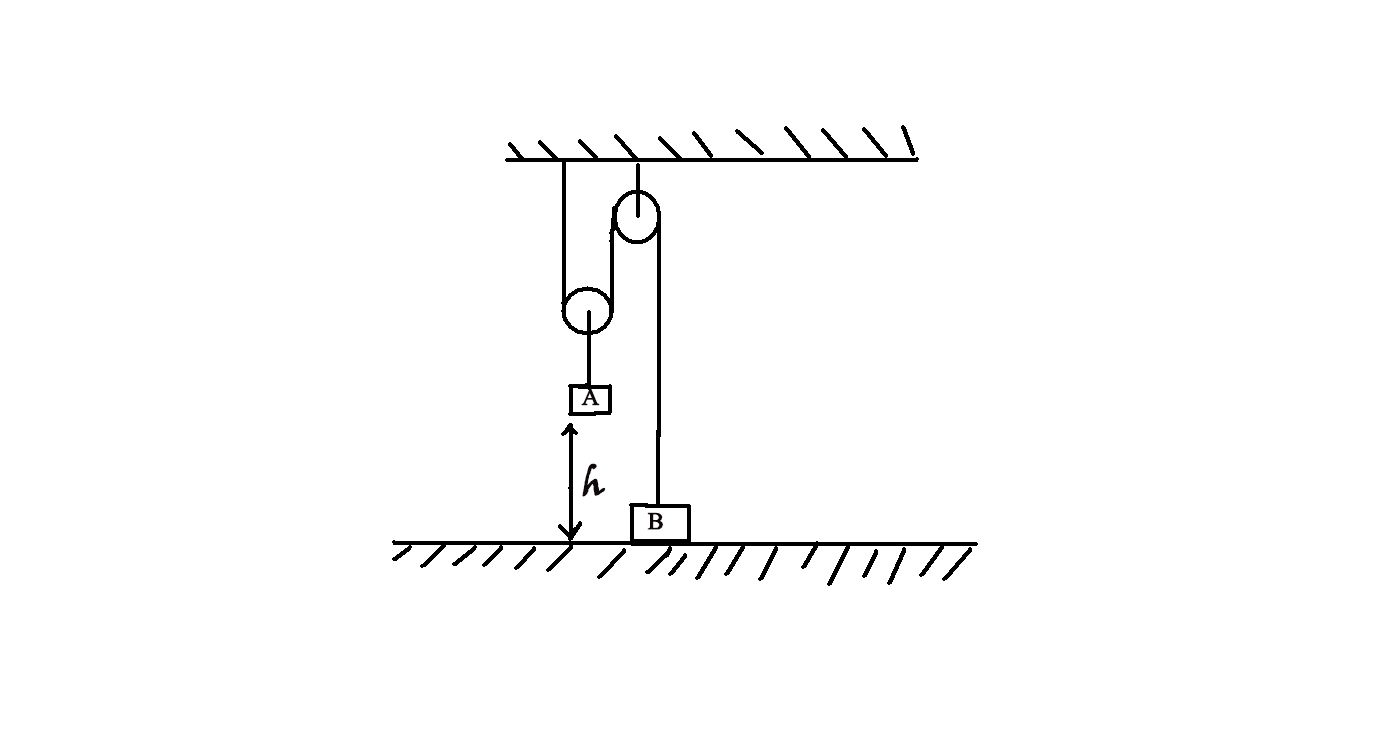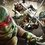# pulley problemIn the arrangement shown in figure, the mass of the body A is 4 times that of body B. The height h=20 cm . At a certain instant, the body B is released and the system is set in motion. What is the maximum height (in cm), the body B will go up? Assume enough space above B and A sticks to the ground.

Can anyone help me to understand the concept behind pulley with the help of problem6 years, 9 months ago

This discussion board is a place to discuss our Daily Challenges and the math and science related to those challenges. Explanations are more than just a solution — they should explain the steps and thinking strategies that you used to obtain the solution. Comments should further the discussion of math and science.

When posting on Brilliant:

• Use the emojis to react to an explanation, whether you're congratulating a job well done , or just really confused .
• Ask specific questions about the challenge or the steps in somebody's explanation. Well-posed questions can add a lot to the discussion, but posting "I don't understand!" doesn't help anyone.
• Try to contribute something new to the discussion, whether it is an extension, generalization or other idea related to the challenge.

MarkdownAppears as
*italics* or _italics_ italics
**bold** or __bold__ bold
- bulleted- list
• bulleted
• list
1. numbered2. list
1. numbered
2. list
Note: you must add a full line of space before and after lists for them to show up correctly
paragraph 1paragraph 2

paragraph 1

paragraph 2

[example link](https://brilliant.org)example link
> This is a quote
This is a quote
    # I indented these lines
# 4 spaces, and now they show
# up as a code block.

print "hello world"
# I indented these lines
# 4 spaces, and now they show
# up as a code block.

print "hello world"
MathAppears as
Remember to wrap math in $$ ... $$ or $ ... $ to ensure proper formatting.
2 \times 3 $2 \times 3$
2^{34} $2^{34}$
a_{i-1} $a_{i-1}$
\frac{2}{3} $\frac{2}{3}$
\sqrt{2} $\sqrt{2}$
\sum_{i=1}^3 $\sum_{i=1}^3$
\sin \theta $\sin \theta$
\boxed{123} $\boxed{123}$

Sort by:

$Let\quad the\quad displacement\quad of\quad mass\quad B\quad =\quad H\\ Let\quad the\quad tension\quad at\quad mass\quad B\quad =\quad T\\ So,\quad if\quad we\quad continue\quad finding\quad the\quad tension\quad upto\\ A,\quad we\quad end\quad up\quad with\quad 2T\quad at\quad mass\quad A.\\ \\ Now,\quad by\quad Virtual\quad work\quad done,\quad \\ Net\quad work\quad done\quad by\quad the\quad internal\quad forces\quad =\quad 0\\ { Force }_{ Int.A }\times { Displacement }_{ A }+{ Force }_{ Int.B }\times { Displacement }_{ B }=\quad 0\quad \\ 2T\quad \times \quad h\quad +\quad T\quad \times \quad H\quad =\quad 0\\ 2hT\quad =\quad -TH\\ Cancelling\quad T\quad from\quad both\quad sides,\\ H\quad =\quad -2h\\ (-)\quad sign\quad shows\quad that\quad displacement\quad is\quad in\quad opposite\quad direction\\ to\quad h\\ \\ Considering\quad only\quad magnitude,\\ H\quad =\quad 2h\\ H\quad =\quad 2\times 20\\ H\quad =\quad 40cm$

- 6 years, 9 months ago

When block B travels 40 cm then it gains a final velocity v

v^2=2as

v^2=2×g/2×0.4....................40cm in 0.4m

v^2=4

v=2m/s

Then we have to find the distance traveled by block under gravity

u^2/2g=s

4/20=s

0.2=s

0.2m=20 cm

Total distance covered = distance covered by block due to pulley + distance covered by block under gravity

=40+20=60cm

Now see if you have any doubt about the ans.

And thank you for sharing you mechanical work method

- 6 years, 9 months ago

Your answer is wrong because in taking components of force you have made an mistake force on block b is 2T and 4mg in downward direction because of taking on T it will not make any sense because both the tension are in upward direction so if you T only the force acting on block it will be totally wrong

- 6 years, 9 months ago

You are in class 10, right? There is a method in class 11, "The virtual work done method". You may learn about it on internet.

- 6 years, 9 months ago

Yes you are right bro. The answer should be 40cmfig

For A

$4mg-2T=4ma_{1}$...................(1)

For B

$T-mg=ma_{2}$..............................(2)

Also $2a_{1}=a_{2}$.............................(3)

putting this value in equation (2)

$T-mg=2ma_{1}$

Multiplying this equation with 2 yields

$2T-2mg=4ma_{1}$............................(4)

Adding eq. 1 and 4 yields

$2mg=8ma_{1}$

So $a_{1}=\frac{g}{4}$ and $a_{2}=\frac{2g}{4}$

As $S=ut+0.5at^{2}$

So for block A $0.2=\frac{g}{8}t^{2}$

or $t^{2}=\frac{8(0.2)}{g}$

So for block B

$S=0.5(\frac{2g}{4})(\frac{8(0.2)}{g})$=0.4 m=40cm

But I must say @Abhineet Nayyar that your method was the shortest I think.

- 6 years, 9 months ago

Satvik but when block b travel 40 cm it's gains a final velocity v which is 2m/s then block b travels a distance till its velocity is decelerated so after calculation the distance traveled by block b under gravity is 20cm . So the total distance traveled by block b is 20+40=60cm

- 6 years, 9 months ago

Yes Yogesh you are right. I completely missed that and I think @Abhineet Nayyar missed that too. :P

Thank you Yogesh for pointing it.

- 6 years, 9 months ago

Satvik i have misconception about friction can you explain me the way to solve h c verma's book question no. 11 in friction chapter. please give a quick response

- 6 years, 8 months ago

Q.11 of exercise?

- 6 years, 8 months ago

yes!

- 6 years, 8 months ago

You just need to draw FBD of the three blocks separately then use $F=ma$.

Do have problem regarding the direction frictional force?Suppose if the blocks are accelerating towards right. Then friction forces acting on the blocks are towards left.

- 6 years, 8 months ago

see i want to buy fundamentals of physics by halliday so which edition should i buy

- 6 years, 8 months ago

Every editions are basically same. So you can by any edition. I think the latest is $9^{th}$ edition.

- 6 years, 8 months ago

i have bought fundamentals of physics 8th edition but there is a problem . There are no answers of problem written so how would we come to know that we have solved the problem correctly.

And i was going through 42 problem of chapter no 2 i got the ans 0.94 m/s^2 is it correct?

- 6 years, 8 months ago

In that book answers of all question in exercise are not given. Only answer of odd question numbers are given.

- 6 years, 8 months ago

OK

- 6 years, 8 months ago

i wanted to ask you a question that was really means centripetal acceleration? i defines that its acceleration is towards center but , as an example if earth rotates at the centripetal acceleration of 9.8 m/s^2 then will earth exert force to us towards space or towards earth itself ?

- 6 years, 8 months ago

You can find the solutions of this book here

- 6 years, 8 months ago

Simply find the accelerations of A and B. Then find the time (t) that A will require to cover distance 'h' then use equations of motion to find the distance covered by B in time 't'.

- 6 years, 9 months ago

The main problem is I don't know to find acceleration in like this arrangement of pulley

- 6 years, 9 months agofig

Always try to draw the FBDs first.

Can you setup two equations for block A and B using newton's second law?

- 6 years, 9 months ago

I got it thank you . Can you tell me how you post diagram in comment box

- 6 years, 9 months ago

Please refer to this note. I think there is a similar problem on brilliant also.

- 6 years, 9 months ago

I have got that wrong so I asked you. And see I have framed equipment like this F-2T=0 T=F/2 taking block b as system a=T/m a=F/2m Thus acceleration of block A a=F/4m because acceleration of block B is twice than A .is this correct

- 6 years, 9 months ago

Equipment is wrong word there should be eq

- 6 years, 9 months ago

I don't understand what is 'F' in your equation.

Refer to diagram:

For Block A

$4mg-2T=4ma$............(1)

For block B

$T-mg=ma_{1}$................(2)

Also $2a=a_{1}$ .................(3)

Now you have 3 equations and 3 variables. Just find the values of a and $a_{1}$

- 6 years, 9 months ago

F refers to the downward force of block A

- 6 years, 9 months ago

So F=4mg.

But you wrote F-2T=0. Block A is not in equilibrium.

- 6 years, 9 months ago

See in first condition before Block B is released they both are in equilibrium

- 6 years, 9 months ago

I have done by different point of view but our result is same 2a=a1

- 6 years, 9 months ago

This eq. is just the part of whole solution. I have made 3 eq earlier. By solving those equations you will get the exact values of the accelerations of the A and B.

- 6 years, 9 months ago

But try to understand I make an sequence of finding T first then the acceleration and the tension in the rope is equal in every period of time so it doesn't matter if I take the period before motion or after

- 6 years, 9 months ago

I think we are approaching to the problem in different ways.

Can you find time period in which block A covered distance 'h'.

- 6 years, 9 months ago

T=0.4s

- 6 years, 9 months ago

i got same too. :)

- 6 years, 9 months ago

We both think in different ways but have same direction

- 6 years, 9 months ago

Yeah! Vector having different magnitude but same direction. :D

- 6 years, 9 months ago

Satvik when do you get time to do all this stuff .when I was in class 10 in didn't get time to do this. What is the board of your school

- 6 years, 9 months ago

I am in CBSE board. Well I have started studying mechanics when I was in class 9. I have studied most part of the mechanics in summer vacations.

- 6 years, 9 months ago

Is CBSE board better than SCC board

- 6 years, 9 months ago

I don't know what is SCC board. What is the board of your school?

- 6 years, 9 months ago

SSC

- 6 years, 9 months ago

satvik i want to ask you about writing comments. In my comments all the words are one behind the other I use enter to start the sentence from new line the but when i click post the words again come one behind the other so how do you write your comment so nicely

- 6 years, 9 months ago

To start a new line you need to press enter key two times.

- 6 years, 9 months ago

Here we have to consider the period in which the blocks are in motion

- 6 years, 9 months ago

@satvik pandey You in class 11th right?

- 6 years, 9 months ago

a comes out to be g/4 and a1=g/2

- 6 years, 9 months ago

Correct. :)

- 6 years, 9 months ago

- 6 years, 9 months ago

No its 60 cm

- 6 years, 9 months ago

- 6 years, 9 months ago

Yes I can

- 6 years, 9 months ago

I and satvik has discussed it's solution below you can refer to that. And if you still have doubt after reference then ask me I will clear the doubt

- 6 years, 9 months ago

Yes its 60cm

- 3 years ago

I agree with you.

- 6 years, 9 months ago

The answer is got to be 40...Wait i'm gonna post a solve!!

- 6 years, 9 months ago

Sorry, Abhineet. I was thinking about the static situation. If A is lowered 20 cm then B will end up at 40 cm higher. I wasn't thinking about the accelerations involved.

- 6 years, 9 months ago

You may also use the Mechanical Energy Conservation Law and have in mind that displacement h of body A will correspond to displacement 2h of body B and that every moment B body speed is twice that of body A. Then you can find B body speed (v) at the instant body A sticks the ground. You will easily get that v^2=2gh. And the maximum height will be 2h+h=3h.

- 6 years, 9 months ago